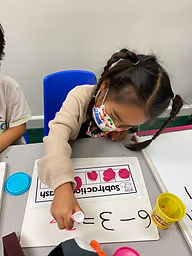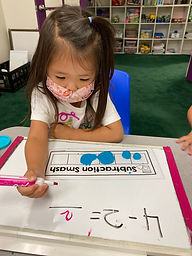## Ms. Brianne

### Target 1​

###### Lesson Type:

New

Number Operation

:

Number System

Identify the number before or after any given number 0 to 10.

###### 1:

Identify the correct number before or after a given number without having to review the entire counting sequence.

###### 2:

Given two numbers, determine which number comes in between.

Kindergarden

###### Vocabulary:

Before, After, Between

Activities:

• Students created their own number lines by writing their numbers in ascending order on pieces of tape.
• Students used their number lines to help them answer questions about the numbers that come before, after, or in between given numbers.### Home Exploration

###### Guiding Questions:## Absent Students:

### Target 2

:

###### 1:

Understand that a larger quantity can be made from two smaller quantities.

###### 2:

Understand that “taking away” makes a smaller quantity.

###### 3:

Represent “taking away” with objects, fingers, drawings, etc.

Kindergarden

###### Vocabulary:

Subtraction, Take Away, Minus

Activities:

• Students played a Subtraction Smash game. They made balls with playdoh to match a given subtraction equation and then smashed the amount being taken away. Then they counted the number left and wrote their answer.
• Students used stickers to represent a subtraction equation and then crossed out to show which ones were being subtracted. Then they counted how many were left and wrote their answer to complete the equation.
• Students practiced solving subtraction problems using their fingers.### Home Exploration

###### Guiding Questions:### Target 3

:

###### 1:

(This is an informal introduction to fractions.)

###### 2:

Distinguish between equal and unequal parts.

###### 3:

Describe if parts are the same or if one is bigger or smaller.

Kindergarden

###### Vocabulary:

Equal, Unequal, Same

Activities:

• Students sorted shapes by whether they showed equal or unequal parts.
• Students used playdoh to create different shapes and then cut them to show equal parts.### Home Exploration